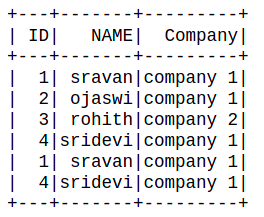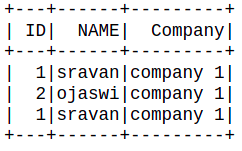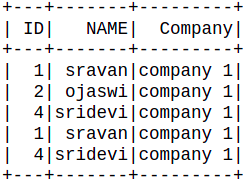Related Articles

# Python PySpark – DataFrame filter on multiple columns

• Last Updated : 14 Sep, 2021

In this article, we are going to filter the dataframe on multiple columns by using filter() and where() function in Pyspark in Python.

Creating Dataframe for demonestration:

## Python3

 `# importing module``import` `pyspark`` ` `# importing sparksession from pyspark.sql module``from` `pyspark.sql ``import` `SparkSession`` ` `# creating sparksession and giving an app name``spark ``=` `SparkSession.builder.appName(``'sparkdf'``).getOrCreate()`` ` `# list  of employee data``data ``=` `[[``1``, ``"sravan"``, ``"company 1"``],``        ``[``2``, ``"ojaswi"``, ``"company 1"``], ``        ``[``3``, ``"rohith"``, ``"company 2"``],``        ``[``4``, ``"sridevi"``, ``"company 1"``],``        ``[``1``, ``"sravan"``, ``"company 1"``],``        ``[``4``, ``"sridevi"``, ``"company 1"``]]`` ` `# specify column names``columns ``=` `[``'ID'``, ``'NAME'``, ``'Company'``]`` ` `# creating a dataframe from the lists of data``dataframe ``=` `spark.createDataFrame(data, columns)`` ` `# display dataframe``dataframe.show()`

Output:## Method 1: Using filter() Method

filter() is used to return the dataframe based on the given condition by removing the rows in the dataframe or by extracting the particular rows or columns from the dataframe. We are going to filter the dataframe on multiple columns. It can take a condition and returns the dataframe.

Syntax:

filter(dataframe.column condition)

Example 1: Conditional operator includes boolean or logical or relational operators.

## Python3

 `# select dataframe where ID less than 3``dataframe.``filter``(dataframe.``ID` `< ``3``).show()`

Output:Example 2: Python program to filter data based on two columns. In this example, we created a pyspark dataframe and select dataframe where ID less than 3 or name is Sridevi

## Python3

 `# select dataframe where ID less than``# 3 or name is sridevi``dataframe.``filter``((dataframe.``ID` `< ``3``) | ``                 ``(dataframe.NAME ``=``=` `'sridevi'``)).show()`

Output:Example 3: Multiple columns filtering

## Python3

 `# select dataframe where ID less than``# 3 or name is sridevi and comapny 1``dataframe.``filter``((dataframe.``ID` `< ``3``) | (``    ``(dataframe.NAME ``=``=` `'sridevi'``) & ``  ``(dataframe.Company ``=``=` `'company 1'``))).show()`

Output:## Method 2: where() method

Where: where is similar to filter() function that is used to return the dataframe based on the given condition by removing the rows in the dataframe or by extracting the particular rows or columns from the dataframe. It can take a condition and returns the dataframe.

where(dataframe.column condition)

Example 1: Python program to filter on multiple columns

## Python3

 `# select dataframe where ID less than``# 3 or name is sridevi and comapny 1``dataframe.where((dataframe.``ID` `< ``3``) | (``    ``(dataframe.NAME ``=``=` `'sridevi'``) &``  ``(dataframe.Company ``=``=` `'company 1'``))).show()`

Output:Attention geek! Strengthen your foundations with the Python Programming Foundation Course and learn the basics.

To begin with, your interview preparations Enhance your Data Structures concepts with the Python DS Course. And to begin with your Machine Learning Journey, join the Machine Learning – Basic Level Course

My Personal Notes arrow_drop_up